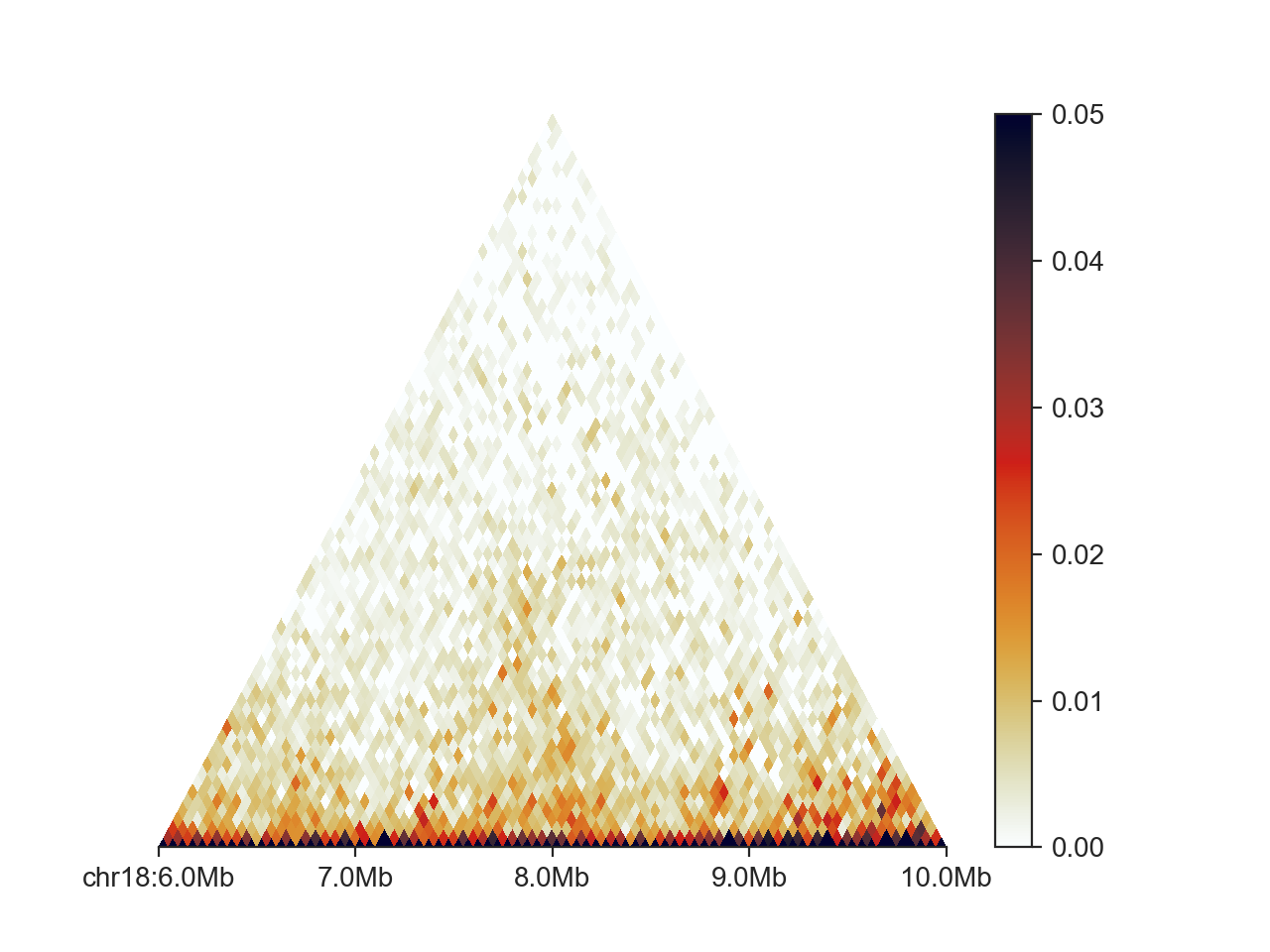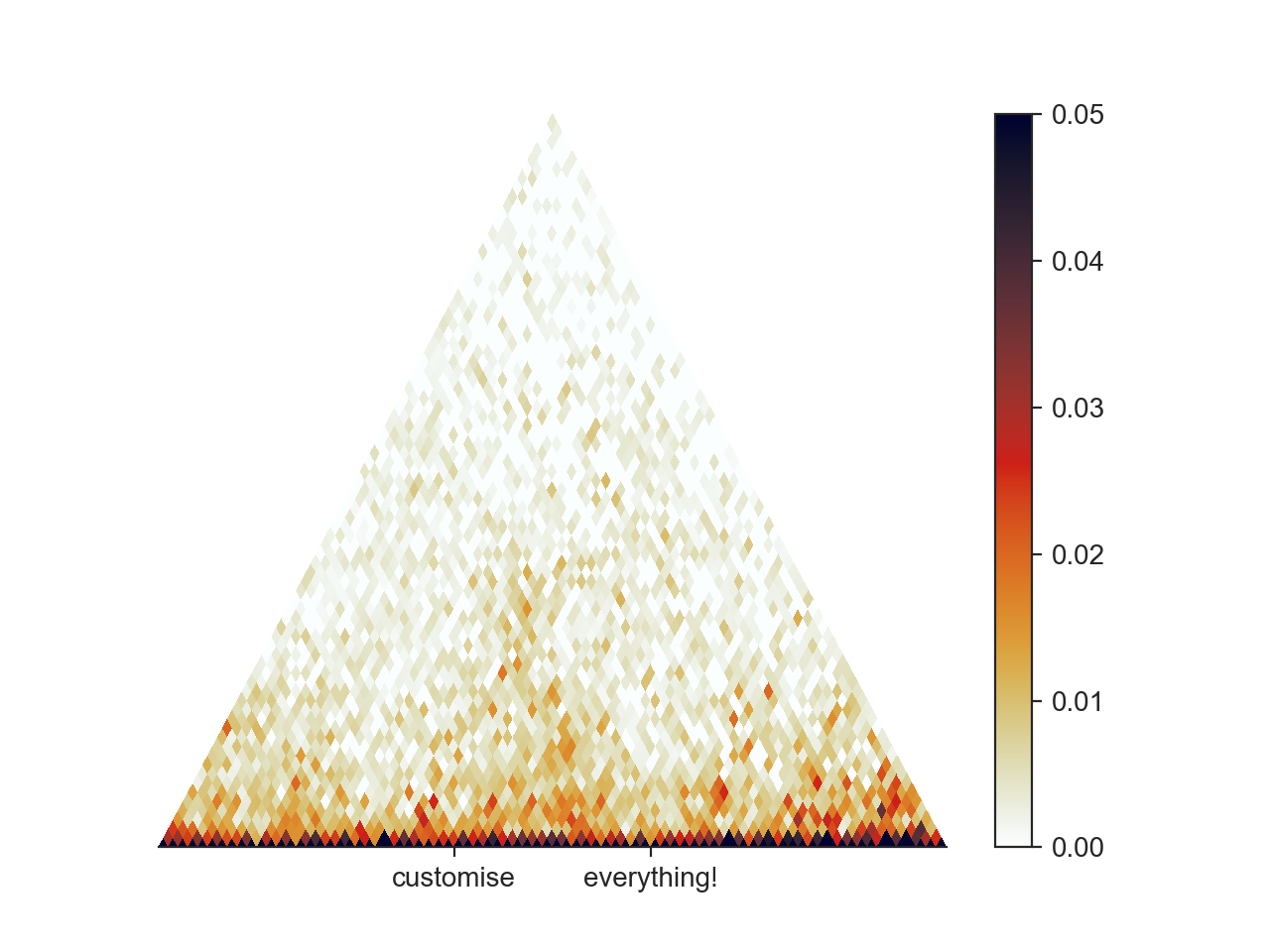# Plotting API basics¶

FAN-C plotting functions are imported in a Python script or console using

```import fanc
import fanc.plotting as fancplot
```

This will provide access to the FAN-C plot types and custom colormaps for matrix visualisation.

For the following sections we will load a `Hic` file for plotting:

```hic = fanc.load("output/hic/binned/fanc_example_50kb.hic")
```

## BasePlotter¶

Each plot type is based on the `BasePlotter` class. It controls the formatting of the X axis in genomic coordinates (kb, mb, etc), and provides a `plot()` function, common to all FAN-C plot types, which accepts genomic interval definitions in the form of strings (`chr18:6mb-8mb`) or `GenomicRegion` objects.

As an example of basic functionality, we will be looking at the `TriangularMatrixPlot`:

```hp = fancplot.TriangularMatrixPlot(hic, vmax=0.05)
hp.plot('chr18:6mb-10mb')
hp.show()
```The first line sets up the triangular matrix plot parameters. Except for `vmax` we have kept the defaults. The second line is where the actual plotting to a genomic region happens and the plot components are assembled. The final line simply opens an interactive plotting window.

To save the plot to file, you can use `hp.save('/path/to/file.png')`.

You can control the formatting of the X axis using a number of different parameters. `draw_ticks` can be set to `False` to remove the major (and minor) tick marks at major genomic locations. `draw_major_ticks` and `draw_minor_ticks` control the drawing of major and minor ticks, respectively, in the same manner. `draw_minor_ticks` is `False` by default. To display a small legend in the bottom right of the plot that shows the distance between major ticks, between minor ticks, and the entire plotting range, set `draw_tick_legend` to True. You can remove the chromosome label at the first location by setting `draw_chromosome_label` to `False`. You can invert the X axis (and the plot) by setting `invert_x` to `True`, or remove the X axis entirely by setting `draw_x_axis` to `False`.

## Using dedicated Matplotlib Axes¶

For the highest level of control over your plot, you can build your figures on top of Matplotlib. This enables you to modify each aspect of the plot after it has been generated. To start, import matplotlib:

```import matplotlib.pyplot as plt
```

And use the `ax` argument of the FAN-C plot to supply it with a custom axis.

```fig, ax = plt.subplots()
hp = fancplot.TriangularMatrixPlot(hic, vmax=0.05, ax=ax)
hp.plot('chr18:6mb-10mb')
ax.set_xticks([7500000, 8500000])
ax.set_xticklabels(['customise', 'everything!'])
hp.show()
```Matplotlib offers an incredible amount of customisation and plotting options, which are too numerous to cover here, but we encourage you to study the Matplotlib documentation for getting your plots to look perfect!